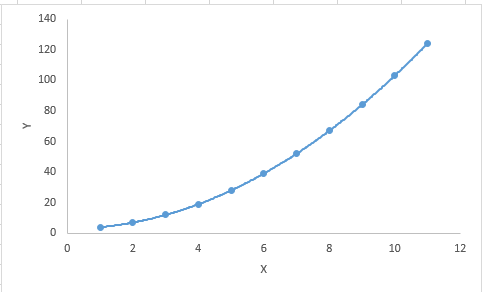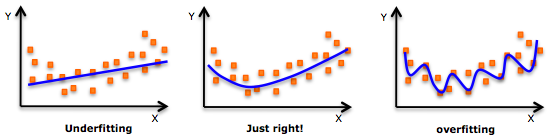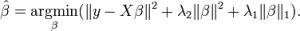# What is Polynomial , Stepwise and ElasticNet Regression?

## Polynomial Regression

A regression equation is a polynomial regression equation if the power of independent variable is more than 1. The equation below represents a polynomial equation:

y=a+b*x^2

In this regression technique, the best fit line is not a straight line. It is rather a curve that fits into the data points.#### Important Points:

• While there might be a temptation to fit a higher degree polynomial to get lower error, this can result in over-fitting. Always plot the relationships to see the fit and focus on making sure that the curve fits the nature of the problem. Here is an example of how plotting can help:• Especially look out for curve towards the ends and see whether those shapes and trends make sense. Higher polynomials can end up producing wierd results on extrapolation.

## Stepwise Regression

This form of regression is used when we deal with multiple independent variables. In this technique, the selection of independent variables is done with the help of an automatic process, which involves no human intervention.

This feat is achieved by observing statistical values like R-square, t-stats and AIC metric to discern significant variables. Stepwise regression basically fits the regression model by adding/dropping co-variates one at a time based on a specified criterion. Some of the most commonly used Stepwise regression methods are listed below:

• Standard stepwise regression does two things. It adds and removes predictors as needed for each step.
• Forward selection starts with most significant predictor in the model and adds variable for each step.
• Backward elimination starts with all predictors in the model and removes the least significant variable for each step.

The aim of this modeling technique is to maximize the prediction power with minimum number of predictor variables. It is one of the method to handle higher dimensionality of data set.

## ElasticNet Regression

ElasticNet is hybrid of Lasso and Ridge Regression techniques. It is trained with L1 and L2 prior as regularizer. Elastic-net is useful when there are multiple features which are correlated. Lasso is likely to pick one of these at random, while elastic-net is likely to pick both.A practical advantage of trading-off between Lasso and Ridge is that, it allows Elastic-Net to inherit some of Ridge’s stability under rotation.

#### Important Points:

• It encourages group effect in case of highly correlated variables
• There are no limitations on the number of selected variables
• It can suffer with double shrinkage